# Title 40

## SECTION 1065.655

### 1065.655 Chemical balances of fuel, intake air, and exhaust.

§ 1065.655 Chemical balances of fuel, intake air, and exhaust.

(a) General. Chemical balances of fuel, intake air, and exhaust may be used to calculate flows, the amount of water in their flows, and the wet concentration of constituents in their flows. With one flow rate of either fuel, intake air, or exhaust, you may use chemical balances to determine the flows of the other two. For example, you may use chemical balances along with either intake air or fuel flow to determine raw exhaust flow. Note that chemical balance calculations require measured values for the flow rate of diesel exhaust fluid, if applicable.

(b) Procedures that require chemical balances. We require chemical balances when you determine the following:

(1) A value proportional to total work, W , when you choose to determine brake-specific emissions as described in § 1065.650(f).

(2) Raw exhaust molar flow rate either from measured intake air molar flow rate or from fuel mass flow rate as described in paragraph (f) of this section.

(3) Raw exhaust molar flow rate from measured intake air molar flow rate and dilute exhaust molar flow rate, as described in paragraph (g) of this section.

(4) The amount of water in a raw or diluted exhaust flow, χH2Oexh, when you do not measure the amount of water to correct for the amount of water removed by a sampling system. Correct for removed water according to § 1065.659.

(5) The calculated total dilution air flow when you do not measure dilution air flow to correct for background emissions as described in § 1065.667(c) and (d).

(c) Chemical balance procedure. The calculations for a chemical balance involve a system of equations that require iteration. We recommend using a computer to solve this system of equations. You must guess the initial values of up to three quantities: The amount of water in the measured flow, xH2Oexh, fraction of dilution air in diluted exhaust, xdil/exh, and the amount of products on a C1 basis per dry mole of dry measured flow, xCcombdry. You may use time-weighted mean values of combustion air humidity and dilution air humidity in the chemical balance; as long as your combustion air and dilution air humidities remain within tolerances of ±0.0025 mol/mol of their respective mean values over the test interval. For each emission concentration, χ, and amount of water, xH2Oexh, you must determine their completely dry concentrations, xdry and xH2Oexhdry. You must also use your fuel mixture's atomic hydrogen-to-carbon ratio, a, oxygen-to-carbon ratio, b, sulfur-to-carbon ratio, g, and nitrogen-to-carbon ratio, d, you may optionally account for diesel exhaust fluid (or other fluids injected into the exhaust), if applicable. You may calculate a, b, g, and d; based on measured fuel and diesel exhaust fluid composition or you may use default values as described in paragraph (e) of this section. Use the following steps to complete a chemical balance:

(1) Convert your measured concentrations such as, xCO2meas, xNOmeas, and xH2Oint, to dry concentrations by dividing them by one minus the amount of water present during their respective measurements; for example: xH2OxCO2meas, xH2OxNOmeas, and xH2Oint. If the amount of water present during a “wet” measurement is the same as the unknown amount of water in the exhaust flow, xH2Oexh, iteratively solve for that value in the system of equations. If you measure only total NOX and not NO and NO2 separately, use good engineering judgment to estimate a split in your total NOX concentration between NO and NO2 for the chemical balances. For example, if you measure emissions from a stoichiometric spark-ignition engine, you may assume all NOX is NO. For a compression-ignition engine, you may assume that your molar concentration of NOX, xNOx, is 75% NO and 25% NO2. For NO2 storage aftertreatment systems, you may assume xNOx is 25% NO and 75% NO2. Note that for calculating the mass of NOX emissions, you must use the molar mass of NO2 for the effective molar mass of all NOX species, regardless of the actual NO2 fraction of NOX.

(2) Enter the equations in paragraph (c)(4) of this section into a computer program to iteratively solve for xH2Oexh, xCcombdry, and xdil/exh. Use good engineering judgment to guess initial values for xH2Oexh, xCcombdry, and xdil/exh. We recommend guessing an initial amount of water that is about twice the amount of water in your intake or dilution air. We recommend guessing an initial value of xCcombdry as the sum of your measured CO2, CO, and THC values. We also recommend guessing an initial xdil/exh between 0.75 and 0.95, such as 0.8. Iterate values in the system of equations until the most recently updated guesses are all within ±1% of their respective most recently calculated values.

(3) Use the following symbols and subscripts in the equations for performing the chemical balance calculations in this paragraph (c):

xdil/exh = amount of dilution gas or excess air per mole of exhaust. xH2Oexh = amount of H2O in exhaust per mole of exhaust. xCcombdry = amount of carbon from fuel in the exhaust per mole of dry exhaust. xH2dry = amount of H2 in exhaust per amount of dry exhaust. KH2Ogas = water-gas reaction equilibrium coefficient. You may use 3.5 or calculate your own value using good engineering judgment. xH2Oexhdry = amount of H2O in exhaust per dry mole of dry exhaust. xprod/intdry = amount of dry stoichiometric products per dry mole of intake air. xdil/exhdry = amount of dilution gas and/or excess air per mole of dry exhaust. xint/exhdry = amount of intake air required to produce actual combustion products per mole of dry (raw or diluted) exhaust. xraw/exhdry = amount of undiluted exhaust, without excess air, per mole of dry (raw or diluted) exhaust. xO2int = amount of intake air O2 per mole of intake air. xCO2intdry = amount of intake air CO2 per mole of dry intake air. You may use χCO2intdry = 375 µmol/mol, but we recommend measuring the actual concentration in the intake air. xH2Ointdry = amount of intake air H2O per mole of dry intake air. xCO2int = amount of intake air CO2 per mole of intake air. xCO2dil = amount of dilution gas CO2 per mole of dilution gas. xCO2dildry = amount of dilution gas CO2 per mole of dry dilution gas. If you use air as diluent, you may use χCO2dildry = 375 µmol/mol, but we recommend measuring the actual concentration in the intake air. xH2Odildry = amount of dilution gas H2O per mole of dry dilution gas. xH2Odil = amount of dilution gas H2O per mole of dilution gas. x[emission]meas = amount of measured emission in the sample at the respective gas analyzer. x[emission]dry = amount of emission per dry mole of dry sample. xH2O[emission]meas = amount of H2O in sample at emission-detection location. Measure or estimate these values according to § 1065.145(e)(2). xH2Oint = amount of H2O in the intake air, based on a humidity measurement of intake air. a = atomic hydrogen-to-carbon ratio of the fuel (or mixture of test fuels) and any injected fluids. b = atomic oxygen-to-carbon ratio of the fuel (or mixture of test fuels) and any injected fluids. g = atomic sulfur-to-carbon ratio of the fuel (or mixture of test fuels) and any injected fluids. d = atomic nitrogen-to-carbon ratio of the fuel (or mixture of test fuels) and any injected fluids.

(4) Use the following equations to iteratively solve for xdil/exh, xH2Oexh, and xCcombdry: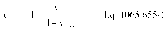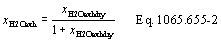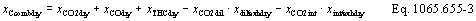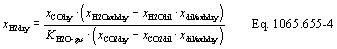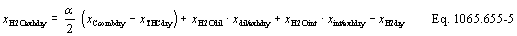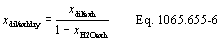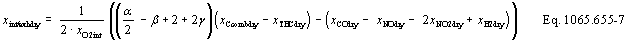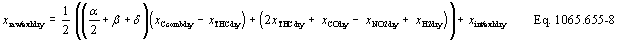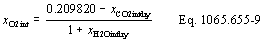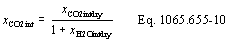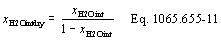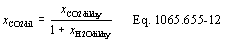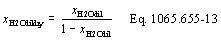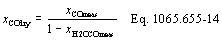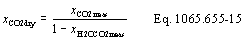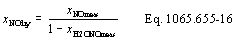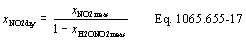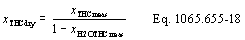(5) The following example is a solution for xdil/exh,x, xH2Oexh, and xCcombdry using the equations in paragraph (c)(4) of this section: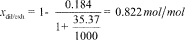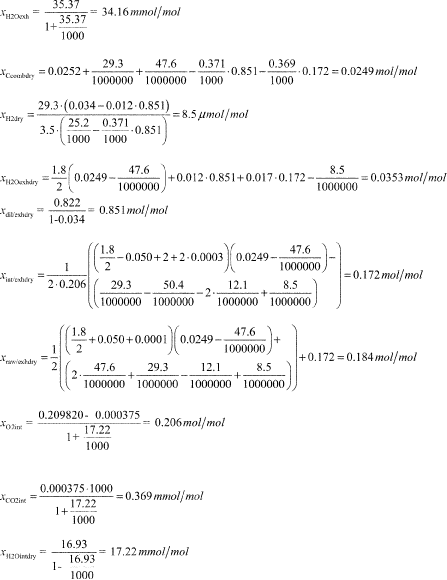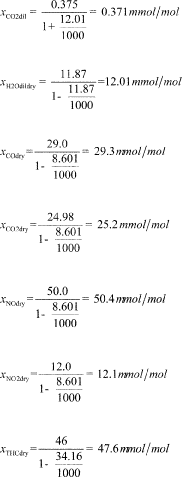a = 1.8 b = 0.05 g = 0.0003 d = 0.0001

(d) Carbon mass fraction of fuel. Determine carbon mass fraction of fuel, wC, based on the fuel properties as determined in paragraph (e) of this section, accounting for diesel exhaust fluid's contribution to a, b, g, and d, or that of any other fluid injected into the exhaust, if applicable. Calculate wC using the following equation: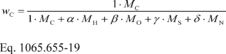Where: wC = carbon mass fraction of the fuel (or mixture of test fuels) and any injected fluids. MC = molar mass of carbon. a = atomic hydrogen-to-carbon ratio of the fuel (or mixture of test fuels) and any injected fluids. MH = molar mass of hydrogen. b = atomic oxygen-to-carbon ratio of the fuel (or mixture of test fuels) and any injected fluids. MO = molar mass of oxygen. g = atomic sulfur-to-carbon ratio of the fuel (or mixture of test fuels) and any injected fluids. MS = molar mass of sulfur. d = atomic nitrogen-to-carbon ratio of the fuel (or mixture of test fuels) and any injected fluids. MN = molar mass of nitrogen. Example: a = 1.8 b = 0.05 g = 0.0003 d = 0.0001 MC = 12.0107 MH = 1.00794 MO = 15.9994 MS = 32.065 MN = 14.0067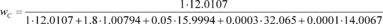wC = 0.8206

(e) Fuel and diesel exhaust fluid composition. Determine fuel and diesel exhaust fluid composition represented by a, b, g, and d as described in this paragraph (e). When using measured fuel or diesel exhaust fluid properties, you must determine values for a and b; in all cases. If you determine compositions based on measured values and the default value listed in Table 1 of this section is zero, you may set g and d to zero; otherwise determine g and d (along with a and b) based on measured values. Determine elemental mass fractions and values for a, b, g, and d as follows:

(1) For liquid fuels, use the default values for a, b, g, and d in Table 1 of this section or determine mass fractions of liquid fuels for calculation of a, b, g, and d as follows:

(i) Determine the carbon and hydrogen mass fractions according to ASTM D5291 (incorporated by reference in § 1065.1010). When using ASTM D5291 to determine carbon and hydrogen mass fractions of gasoline (with or without blended ethanol), use good engineering judgment to adapt the method as appropriate. This may include consulting with the instrument manufacturer on how to test high-volatility fuels. Allow the weight of volatile fuel samples to stabilize for 20 minutes before starting the analysis; if the weight still drifts after 20 minutes, prepare a new sample. Retest the sample if the carbon, hydrogen, and oxygen mass fractions do not add up to a total mass of 100 ±0.5%; if you do not measure oxygen, you may assume it has a zero concentration for this specification.

(ii) Determine oxygen mass fraction of gasoline (with or without blended ethanol) according to ASTM D5599 (incorporated by reference in § 1065.1010). For all other liquid fuels, determine the oxygen mass fraction using good engineering judgment.

(iii) Determine the nitrogen mass fraction according to ASTM D4629 or ASTM D5762 (incorporated by reference in § 1065.1010) for all liquid fuels. Select the correct method based on the expected nitrogen content.

(iv) Determine the sulfur mass fraction according to subpart H of this part.

(2) For gaseous fuels and diesel exhaust fluid, use the default values for a, b, g, and d in Table 1 of this section, or use good engineering judgment to determine those values based on measurement.

(3) For nonconstant fuel mixtures, you must account for the varying proportions of the different fuels. This generally applies for dual-fuel engines, but it also applies if diesel exhaust fluid is injected in a way that is not strictly proportional to fuel flow. Account for these varying concentrations either with a batch measurement that provides averaged values to represent the test interval, or by analyzing data from continuous mass rate measurements. Application of average values from a batch measurement generally applies to situations where one fluid is a minor component of the total fuel mixture, for example dual-fuel engines with diesel pilot injection, where the diesel pilot fuel mass is less than 5% of the total fuel mass and diesel exhaust fluid injection; consistent with good engineering judgment.

(4) Calculate a, b, g, and d using the following equations: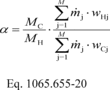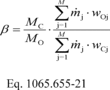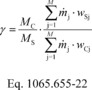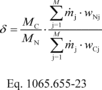Where: M = total number of fuels and injected fluids over the duty cycle. j = an indexing variable that represents one fuel or injected fluid, starting with j = 1. m j = the mass flow rate of the fuel or any injected fluid j. For applications using a single fuel and no DEF fluid, set this value to 1. For batch measurements, divide the total mass of fuel over the test interval duration to determine a mass rate. WHj = hydrogen mass fraction of fuel or any injected fluid j. WCj = carbon mass fraction of fuel or any injected fluid j. WOj = oxygen mass fraction of fuel or any injected fluid j. WSj = sulfur mass fraction of fuel or any injected fluid j. WNj = nitrogen mass fraction of fuel or any injected fluid j. Example: N = 1 j = 1 m j = 1 WHj = 0.1239 WCj = 0.8206 WOj = 0.0547 WSj = 0.00066 WNj = 0.000095 MC = 12.0107 MH = 1.00794 MO = 15.9994 MS = 32.065 MN = 14.0067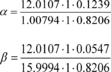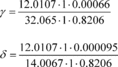a = 1.799 b = 0.05004 g = 0.0003012 d = 0.0001003

Table 1 of § 1065.655 - Default Values of a, b, g, d, and WC

Fuel or injected fluid Atomic hydrogen,
oxygen, sulfur, and
nitrogen-to-carbon ratios
CHαOβSγNδ
Carbon mass
fraction, WC
g/g
Gasoline CH1.85O0S0N0 0.866
E10 Gasoline CH1.92O0.03S0N0 0.833
E15 Gasoline CH1.95O0.05S0N0 0.817
E85 Gasoline CH2.73O0.38S0N0 0.576
E100 Ethanol CH3O0.5S0N0 0.521
M100 Methanol CH4O1S0N0 0.375
#1 Diesel CH1.93O0S0N0 0.861
#2 Diesel CH1.80O0S0N0 0.869
Liquefied petroleum gas CH2.64O0S0N0 0.819
Natural gas CH3.78 O0.016S0N0 0.747
Residual fuel blends Must be determined by measured fuel properties as described in paragraph (d)(1) of this section.
Diesel exhaust fluid CH17.85O7.92S0N2 0.065

(f) Calculated raw exhaust molar flow rate from measured intake air molar flow rate or fuel mass flow rate. You may calculate the raw exhaust molar flow rate from which you sampled emissions, n exh, based on the measured intake air molar flow rate, n int, or the measured fuel mass flow rate, m fuel, and the values calculated using the chemical balance in paragraph (c) of this section. The chemical balance must be based on raw exhaust gas concentrations. Solve for the chemical balance in paragraph (c) of this section at the same frequency that you update and record or n int or m fuel. For laboratory tests, calculating raw exhaust molar flow rate using measured fuel mass flow rate is valid only for steady-state testing. See § 1065.915(d)(5)(iv) for application to field testing.

(1) Crankcase flow rate. If engines are not subject to crankcase controls under the standard-setting part, you may calculate raw exhaust flow based on n int or m fuel using one of the following:

(i) You may measure flow rate through the crankcase vent and subtract it from the calculated exhaust flow.

(ii) You may estimate flow rate through the crankcase vent by engineering analysis as long as the uncertainty in your calculation does not adversely affect your ability to show that your engines comply with applicable emission standards.

(iii) You may assume your crankcase vent flow rate is zero.

(2) Intake air molar flow rate calculation. Calculate n exh based on n int using the following equation: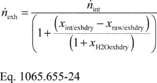Where: n exh = raw exhaust molar flow rate from which you measured emissions. n int = intake air molar flow rate including humidity in intake air. Example: n int = 3.780 mol/s xint/exhdry = 0.69021 mol/mol xraw/exhdry = 1.10764 mol/mol xH20exhdry = 107.64 mmol/mol = 0.10764 mol/mol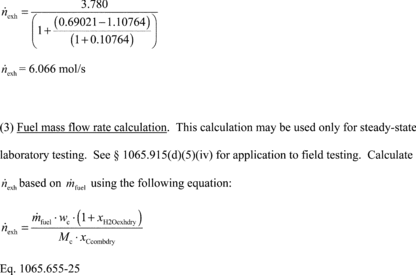(3) Fluid mass flow rate calculation. This calculation may be used only for steady-state laboratory testing. See § 1065.915(d)(5)(iv) for application to field testing. Calculate n exh based on using the following equation: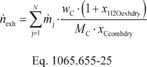Where: n exh = raw exhaust molar flow rate from which you measured emissions. N = total number of fuels and injected fluids over the duty cycle. j = an indexing variable that represents one fuel or injected fluid, starting with j = 1. m j = the mass flow rate of the fuel or any injected fluid j. Example: N = 1 j = 1 m j = 7.559 g/s wC = 0.869 g/g MC = 12.0107 g/mol xCcombdry = 99.87 mmol/mol = 0.09987 mol/mol xH20exhdry = 107.64 mmol/mol = 0.10764 mol/mol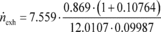n exh = 6.066 mol/s

(g) Calculated raw exhaust molar flow rate from measured intake air molar flow rate, dilute exhaust molar flow rate, and dilute chemical balance. You may calculate the raw exhaust molar flow rate, n exh, based on the measured intake air molar flow rate, n int, the measured dilute exhaust molar flow rate, n dexh, and the values calculated using the chemical balance in paragraph (c) of this section. Note that the chemical balance must be based on dilute exhaust gas concentrations. For continuous-flow calculations, solve for the chemical balance in paragraph (c) of this section at the same frequency that you update and record n int and n dexh. This calculated n exh may be used for the PM dilution ratio verification in § 1065.546; the calculation of dilution air molar flow rate in the background correction in § 1065.667; and the calculation of mass of emissions in § 1065.650(c) for species that are measured in the raw exhaust.

(1) Crankcase flow rate. If engines are not subject to crankcase controls under the standard-setting part, calculate raw exhaust flow as described in paragraph (e)(1) of this section.

(2) Dilute exhaust and intake air molar flow rate calculation. Calculate n exh as follows: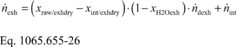Example: n int = 7.930 mol/s xraw/exhdry = 0.1544 mol/mol xint/exhdry = 0.1451 mol/mol xH20/exh = 32.46 mmol/mol = 0.03246 mol/mol n dexh = 49.02 mol/s n exh = (0.1544 − 0.1451) · (1 − 0.03246) · 49.02 + 7.930 = 0.4411 + 7.930 = 8.371 mol/s [73 FR 37331, June 30, 2008, as amended at 73 FR 59334, Oct. 8, 2008; 75 FR 23051, Apr. 30, 2010; 76 FR 57458, Sept. 15, 2011; 79 FR 23799, Apr. 28, 2014; 81 FR 74182, Oct. 25, 2016]